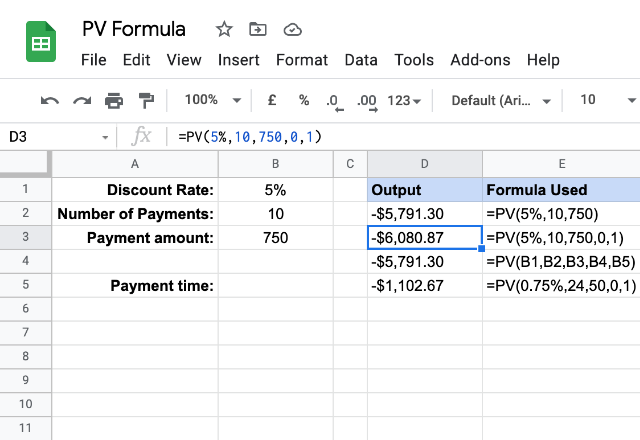# How to use the PV formula in Google SheetsAll of us try and invest our money in some assets. So, we would definitely want to know how much value our future investments stand for, as of today. The PVformula in Google Sheets helps us with just that. Given the periodic payment amount and the interest rate that doesn’t change over time, it calculates the present value of an investment.

### Syntax

PV(rate, number_of_periods, payment_amount, [future_value], [end_or_beginning])

• rate – it is the rate of interest that doesn’t change over a time.
• number_of_periods – is the number of periodic payments that we are going to make.
• payment_amount –  is the constant amount of money that we pay for each period.
• future_value – [OPTIONAL] – is the future value that remains, if at all, after we make the final payment.
• end_or_beginning – [OPTIONAL – 0 by default] – a 0 indicates that we are making the payments at the end of each period. A value of 1 specifies that we are making payments at the beginning of each payment period.

### Usage: PV formula in Google Sheets

Now that we learned the syntax of the PV formula, let’s try our hands on it in practice on the Google Sheets application directly. Please consider the following snapshot.In the first three formulas, we tried to calculate the present value of an investment that is spaced across 10 periods. The interest rate is 5% and the amount we are paying up is 750. While the first and third formulas are essentially representing the same thing, the second formula is a little interesting. All the parameter values in this formula are same, except the last one. So, the value 1 for the last parameter indicates that we are paying up at the end of the period, hence the difference in output.

Please note that this formula remains valid as long as the payment intervals remain spaced evenly. It doesn’t matter if we make the payments monthly or even weekly. It is just that the interest rates vary accordingly. The fourth formula is an example of such calculation, for a monthly based investment series.

#### Note

Functionality wise, we have a close sibling of the PV formula in Google Sheets – the NPV formula. While both of them return the present value of a series of investments, the PV formula is suitable for constant-amount future value cash flows. Whereas the NPV can handle the cash flows even if they are not of a consistent amount.